# Types Of Data Used In Cluster Analysis - Data Mining

## Types Of Data Used In Cluster Analysis Are:

• Interval-Scaled variables
• Binary variables
• Nominal, Ordinal, and Ratio variables
• Variables of mixed types

## Types Of Data Structures

First of all, let us know what types of data structures are widely used in cluster analysis.

We shall know the types of data that often occur in cluster analysis and how to preprocess them for such analysis.

Suppose that a data set to be clustered contains n objects, which may represent persons, houses, documents, countries, and so on.

Main memory-based clustering algorithms typically operate on either of the following two data structures.

Types of data structures in cluster analysis are
• Data Matrix (or object by variable structure)
• Dissimilarity Matrix (or object by object structure)

(Checkout No.1 Data Science Course On Udemy)

## Data Matrix

This represents n objects, such as persons, with p variables (also called measurements or attributes), such as age, height, weight, gender, race and so on. The structure is in the form of a relational table, or n-by-p matrix (n objects x p variables)

The Data Matrix is often called a two-mode matrix since the rows and columns of this represent the different entities.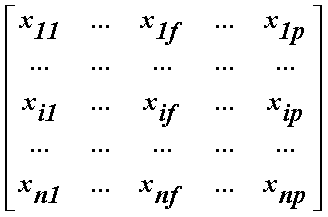## Dissimilarity Matrix

This stores a collection of proximities that are available for all pairs of n objects. It is often represented by a n – by – n table, where d(i,j) is the measured difference or dissimilarity between objects i and j. In general, d(i,j) is a non-negative number that is close to 0 when objects i and j are higher similar or “near” each other and becomes larger the more they differ. Since d(i,j) = d(j,i) and d(i,i) =0, we have the matrix in figure.

This is also called as one mode matrix since the rows and columns of this represent the same entity.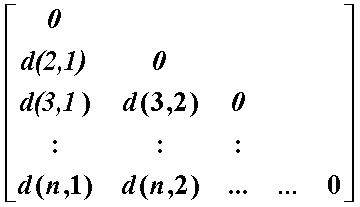## Interval-Scaled Variables

Interval-scaled variables are continuous measurements of a roughly linear scale.

Typical examples include weight and height, latitude and longitude coordinates (e.g., when clustering houses), and weather temperature.

The measurement unit used can affect the clustering analysis. For example, changing measurement units from meters to inches for height, or from kilograms to pounds for weight, may lead to a very different clustering structure.

In general, expressing a variable in smaller units will lead to a larger range for that variable, and thus a larger effect on the resulting clustering structure.

To help avoid dependence on the choice of measurement units, the data should be standardized. Standardizing measurements attempts to give all variables an equal weight.

This is especially useful when given no prior knowledge of the data. However, in some applications, users may intentionally want to give more weight to a certain set of variables than to others.

For example, when clustering basketball player candidates, we may prefer to give more weight to the variable height.

## Binary Variables

A binary variable is a variable that can take only 2 values.

For example, generally, gender variables can take 2 variables male and female.

### Contingency Table For Binary Data

Let us consider binary values 0 and 1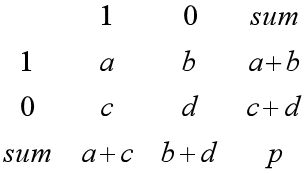Let p=a+b+c+d

Simple matching coefficient (invariant, if the binary variable is symmetric):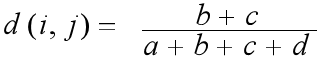Jaccard coefficient (noninvariant if the binary variable is asymmetric):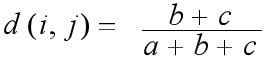## Nominal or Categorical Variables

A generalization of the binary variable in that it can take more than 2 states, e.g., red, yellow, blue, green.

### Method 1: Simple matching

The dissimilarity between two objects i and j can be computed based on the simple matching.

m: Let m be no of matches (i.e., the number of variables for which i and j are in the same state).

p: Let p be total no of variables.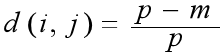### Method 2: use a large number of binary variables

Creating a new binary variable for each of the M nominal states.

## Ordinal Variables

An ordinal variable can be discrete or continuous.

In this order is important, e.g., rank.

It can be treated like interval-scaled

By replacing xif by their rank,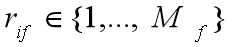By mapping the range of each variable onto [0, 1] by replacing the i-th object in the f-th variable by,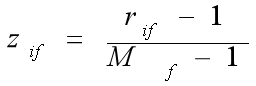Then compute the dissimilarity using methods for interval-scaled variables.

## Ratio-Scaled Intervals

Ratio-scaled variable: It is a positive measurement on a nonlinear scale, approximately at an exponential scale, such as Ae^Bt or A^e-Bt.

Methods:
• First, treat them like interval-scaled variables — not a good choice! (why?)
• Then apply logarithmic transformation i.e.y = log(x)
• Finally, treat them as continuous ordinal data treat their rank as interval-scaled.

## Variables Of Mixed Type

A database may contain all the six types of variables
symmetric binary, asymmetric binary, nominal, ordinal, interval, and ratio.

And those combinedly called as mixed-type variables.

## Summary

Data Types in Cluster Analysis
• Interval-Scaled variables
• Binary variables
• Nominal, Ordinal, and Ratio variables
• Variables of mixed types

Read also -> Clustering In Data Mining

Subscribe us for more content on Data.